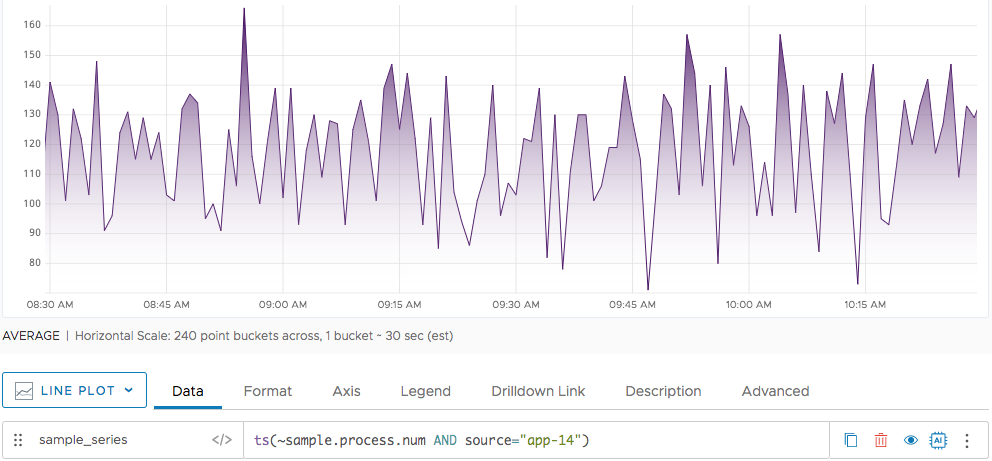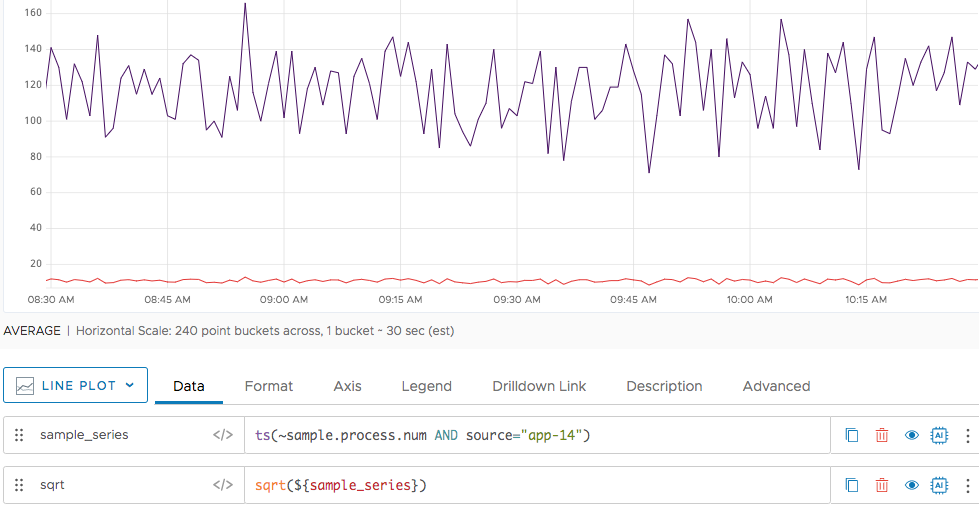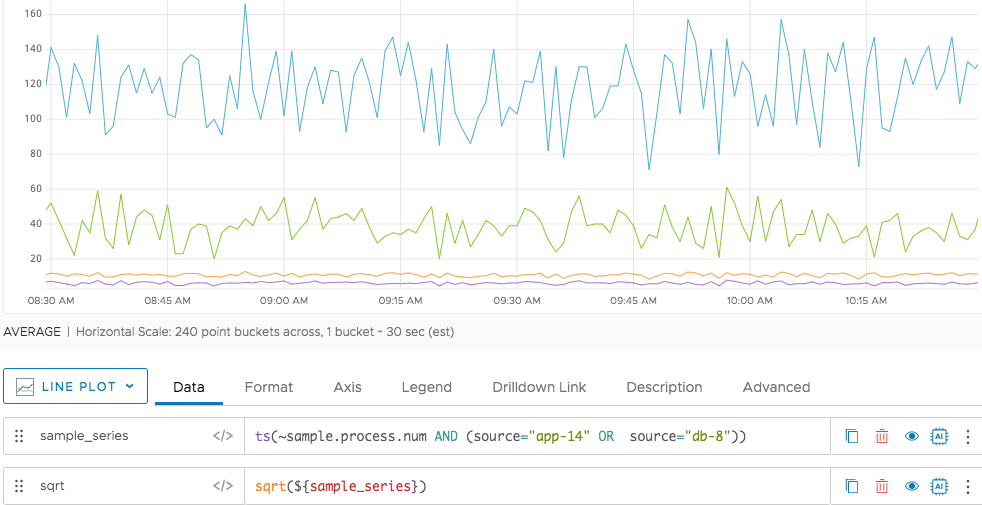Reference to the sqrt() function

## Summary

``````sqrt(<tsExpression>)
``````

Returns the square root of each data value in the time series described by the expression.

## Parameters

ParameterDescription
tsExpression Expression describing the time series of data values to find the square root of.

## Description

The `sqrt()` function produces data points by taking the square root of the data points returned by the input expression.

• If `tsExpression` is a constant, then `sqrt()` returns the square root of that constant.
• If `tsExpression` describes one or more time series, then `sqrt()` returns a new time series for each input time series. Each value in a new time series is obtained by taking the square root of the value of the corresponding point in the input time series.

## Examples

In this example, your chart shows the values in the metric `~sample.process.num` for a single source.And here we see the result of applying the `sqrt` function to the expression.Here we see what happens when the input expression returns data points for 2 sources, app-14 and db-8. Notice that `sqrt` returns two time series, one for each series returned by the expression.